# C program to count characters, spaces, tabs, and newlines in a file

Before starting to write a program in C that counts the total number of characters, spaces, tabs, and newlines present in any file inside the current directory. Here "current directory" means the directory where your C program files are getting saved.

Now let's create a text file named codescracker.txt and write the following line:

```hello	everyone
i am	a file
this is codescracker
there are total of 4 lines present here```

Now put this file inside the current directory.

Here's a screenshot of the file codescracker.txt, which contains all of the above text (four lines). In this screenshot, you can also see that the file is saved inside the current directory: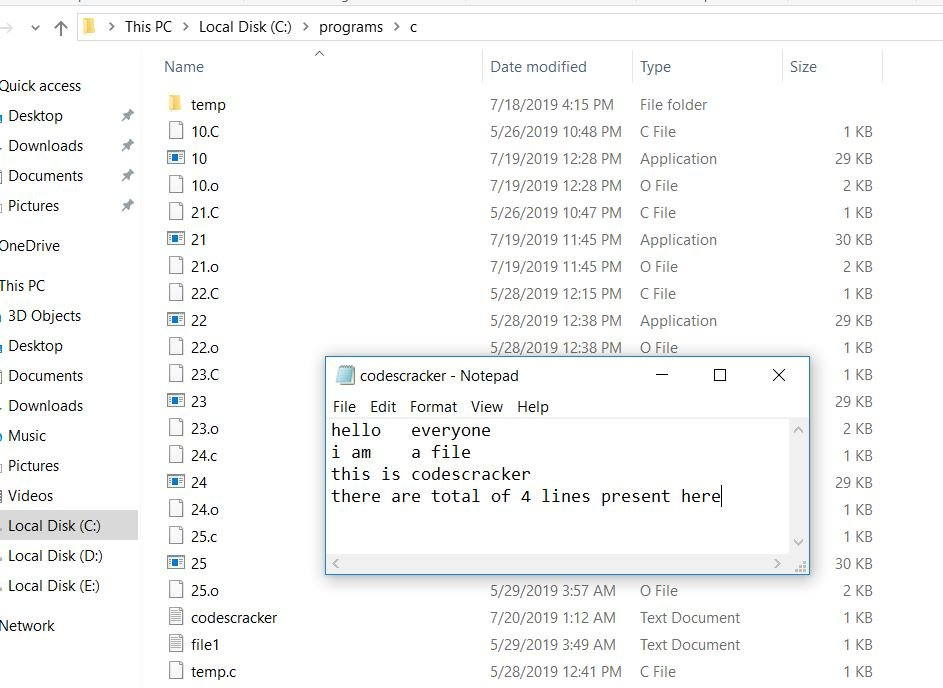Now here is the program that counts characters, spaces, tabs, and newlines present inside the file that was created earlier, named codescracker.txt. You can also create any file and count these things for that file. Or you can also enter any filename that already exists inside the current directory:

```#include<stdio.h>
#include<conio.h>
int main()
{
FILE *fp;
char ch, fname;
int noOfChar=0, noOfSpace=0, noOfTab=0, noOfNewline=0;
printf("Enter file name with extension: ");
gets(fname);
fp = fopen(fname, "r");
while(fp)
{
ch = fgetc(fp);
if(ch==EOF)
break;
noOfChar++;
if(ch==' ')
noOfSpace++;
if(ch=='\t')
noOfTab++;
if(ch=='\n')
noOfNewline++;
}
fclose(fp);
printf("\nNumber of characters = %d", noOfChar);
printf("\nNumber of spaces = %d", noOfSpace);
printf("\nNumber of tabs = %d", noOfTab);
printf("\nNumber of newline = %d", noOfNewline);
getch();
return 0;
}```

As the above program is written in the Code::Blocks IDE, here is the sample run after it has been successfully built and run. This is the first screenshot:Now enter the name of that file with the appropriate extension that has been created in the current directory and press the enter key. If you will also create the same file with the same content, then here is the second screenshot of the sample run that you will also get on your output screen: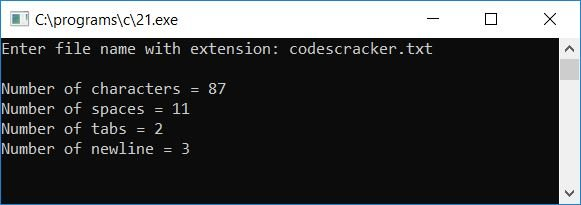As you can see, the program shows the total number of characters, spaces, tabs, and newlines present in the file codescracker.txt.

You can also type the name of the file in which your current program is written. In my case, the name of the program is 21.c. Let's type 21.c. and find out what will happen: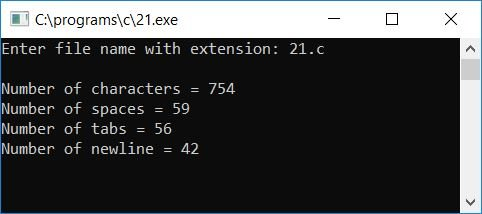As you can see, the program also prints the total number of characters, tabs, spaces, and newlines present inside the program that you have written and saved.

Note: Before typing your current program name as a filename, be sure that you have saved your program.

Here are some of the main steps used in the above program:

1. Get the file name as input from the user at run-time. Always ask the user to enter the filename along with its extension, say, codescracker.txt. Here ".txt" is the extension of the file.
2. Open that file with the fopen() function to read the content contained within it.
3. Create a while loop and run this loop until the file pointer goes to the end of the file.
4. Get the character from the file using the fgetc() function and initialize it to the ch variable.
5. Check whether ch is equal to the end of the file; if it is, then break out of the loop; otherwise, continue to the next statement.
6. First, increment the value of the variable that is responsible for character counting. Because everything inside the file is character.
7. And then check whether the character variable is equal to space; if it is, then increment the value of the variable that is responsible for space counting.
8. In a similar way, increment the value of the tab and newline counting variables, if found.
9. In this way, you have a total of four variables, namely, noOfChar, noOfSpace, noOfTab, and noOfNewline.
10. All these four variables hold the total number of characters, spaces, tabs, and newlines present inside the given file.
11. After breaking out of the loop, never forget to close the file pointer using the fclose() function.
12. Finally, print out the value of all four variables.

You can also add some more lines of coding to count extra things like vowels, numbers, etc. Let's create another program with some extra lines of code along with its sample run. The question is: write a program in C to count the following things inside any file entered by the user at run-time:

1. Total Number of Characters
2. Total Number of Spaces
3. Total Number of Tabs
4. Total Number NewLines
5. Total Number Vowels
6. Total Number of Consonants
7. Total Number of Numbers

The program given below is the answer to this question.

```#include<stdio.h>
#include<conio.h>
int main()
{
FILE *fp;
char ch, fname;
int noOfChar=0, noOfSpace=0, noOfTab=0, noOfNewline=0;
int noOfVowel=0, noOfConsonant=0, noOfNumber=0;
printf("Enter file name with extension: ");
gets(fname);
fp = fopen(fname, "r");
while(fp)
{
ch = fgetc(fp);
if(ch==EOF)
break;
noOfChar++;
if(ch>=65 && ch<=90)
{
noOfConsonant++;
if(ch=='A' || ch=='E' || ch=='I' || ch=='O' || ch=='U')
noOfVowel++;
}
if(ch>=97 && ch<=122)
{
noOfConsonant++;
if(ch=='a' || ch=='e' || ch=='i' || ch=='o' || ch=='u')
noOfVowel++;
}
if(ch>=48 && ch<=57)
noOfNumber++;
if(ch==' ')
noOfSpace++;
if(ch=='\t')
noOfTab++;
if(ch=='\n')
noOfNewline++;
}
fclose(fp);
printf("\nTotal Number of Characters = %d", noOfChar);
printf("\nTotal Number of Spaces = %d", noOfSpace);
printf("\nTotal Number of Tabs = %d", noOfTab);
printf("\nTotal Number NewLines = %d", noOfNewline);
printf("\nTotal Number Vowels = %d", noOfVowel);
printf("\nTotal Number of Consonants = %d", noOfConsonant);
printf("\nTotal Number of Numbers = %d", noOfNumber);
getch();
return 0;
}```

After successfully building and running, here is the first screenshot of the sample run of the above program: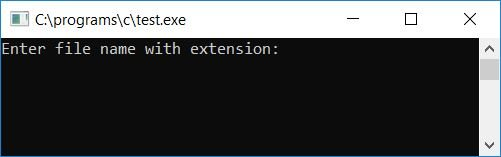Now enter any file name. Let's enter the current filename, where we have written and saved our current program, which is test.c in this case, and press the ENTER key to see the following output: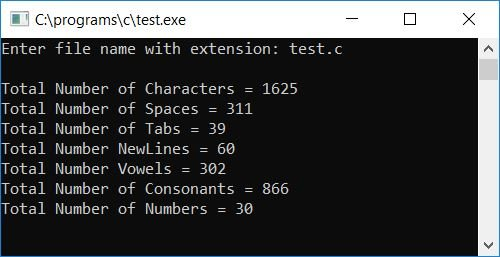C Quiz

« Previous Program Next Program »